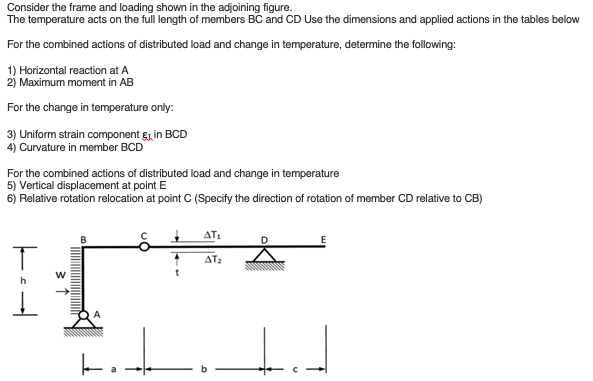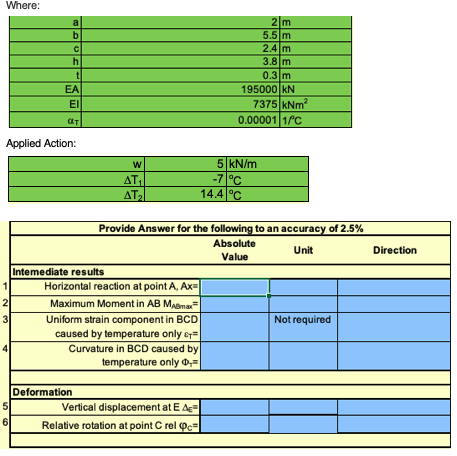# Question Consider the frame and loading shown in the adjoining figure. The temperature acts on the full length of members BC and CD Use the dimensions and applied actions in the tables below For the combined actions of distributed load and change in temperature, determine the following: 1) Horizontal reaction at A 2) Maximum moment in AB For the change in temperature only: 3) Uniform strain component Ex in BCD 4) Curvature in member BCD For the combined actions of distributed load and change in temperature 5) Vertical displacement at point E 6) Relative rotation relocation at point C (Specify the direction of rotation of member CD relative to CB) ΔΤ: B 1 AT2 w I Where: b с h t 2 m 5.5 m 2.4m 3.8 m 0.3 m 195000 KN 7375 kNm? 0.00001 1c ΕΑΙ ΕΙ ат Applied Action: : W ΔΤ, ATAI 5 kN/m -7°C 14.4°C Direction 1 Provide Answer for the following to an accuracy of 2.5% Absolute Unit Value Intemediate results Horizontal reaction at point A, Ax= 2 Maximum Moment in AB Mama 3 Uniform strain component in BCD Not required caused by temperature only & Curvature in BCD caused by temperature only 0,= N Deformation 5 Vertical displacement at E A 6 Relative rotation at point CrelocLJWCAS The Asker · Civil EngineeringTranscribed Image Text: Consider the frame and loading shown in the adjoining figure. The temperature acts on the full length of members BC and CD Use the dimensions and applied actions in the tables below For the combined actions of distributed load and change in temperature, determine the following: 1) Horizontal reaction at A 2) Maximum moment in AB For the change in temperature only: 3) Uniform strain component Ex in BCD 4) Curvature in member BCD For the combined actions of distributed load and change in temperature 5) Vertical displacement at point E 6) Relative rotation relocation at point C (Specify the direction of rotation of member CD relative to CB) ΔΤ: B 1 AT2 w I Where: b с h t 2 m 5.5 m 2.4m 3.8 m 0.3 m 195000 KN 7375 kNm? 0.00001 1c ΕΑΙ ΕΙ ат Applied Action: : W ΔΤ, ATAI 5 kN/m -7°C 14.4°C Direction 1 Provide Answer for the following to an accuracy of 2.5% Absolute Unit Value Intemediate results Horizontal reaction at point A, Ax= 2 Maximum Moment in AB Mama 3 Uniform strain component in BCD Not required caused by temperature only & Curvature in BCD caused by temperature only 0,= N Deformation 5 Vertical displacement at E A 6 Relative rotation at point Creloc
More
Transcribed Image Text: Consider the frame and loading shown in the adjoining figure. The temperature acts on the full length of members BC and CD Use the dimensions and applied actions in the tables below For the combined actions of distributed load and change in temperature, determine the following: 1) Horizontal reaction at A 2) Maximum moment in AB For the change in temperature only: 3) Uniform strain component Ex in BCD 4) Curvature in member BCD For the combined actions of distributed load and change in temperature 5) Vertical displacement at point E 6) Relative rotation relocation at point C (Specify the direction of rotation of member CD relative to CB) ΔΤ: B 1 AT2 w I Where: b с h t 2 m 5.5 m 2.4m 3.8 m 0.3 m 195000 KN 7375 kNm? 0.00001 1c ΕΑΙ ΕΙ ат Applied Action: : W ΔΤ, ATAI 5 kN/m -7°C 14.4°C Direction 1 Provide Answer for the following to an accuracy of 2.5% Absolute Unit Value Intemediate results Horizontal reaction at point A, Ax= 2 Maximum Moment in AB Mama 3 Uniform strain component in BCD Not required caused by temperature only & Curvature in BCD caused by temperature only 0,= N Deformation 5 Vertical displacement at E A 6 Relative rotation at point Creloc